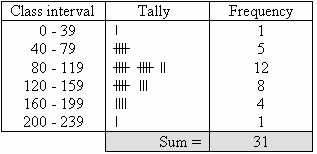Year 8 Interactive Maths - Second Edition

## Frequency and Frequency Tables

The frequency of a particular data value is the number of times the data value occurs.

For example, if four students have a score of 80 in mathematics, and then the score of 80 is said to have a frequency of 4.  The frequency of a data value is often represented by f.

A frequency table is constructed by arranging collected data values in ascending order of magnitude with their corresponding frequencies.

#### Example 5

The marks awarded for an assignment set for a Year 8 class of 20 students were as follows:
6     7     5     7     7     8     7     6     9     7
4     10   6     8     8     9     5     6     4     8

Present this information in a frequency table.

##### Solution:

To construct a frequency table, we proceed as follows:

Step 1:

Construct a table with three columns.  The first column shows what is being arranged in ascending order (i.e. the marks).  The lowest mark is 4.  So, start from 4 in the first column as shown below.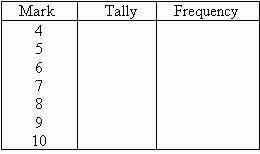Step 2:

Go through the list of marks.  The first mark in the list is 6, so put a tally mark against 6 in the second column.  The second mark in the list is 7, so put a tally mark against 7 in the second column.  The third mark in the list is 5, so put a tally mark against 5 in the third column as shown below.We continue this process until all marks in the list are tallied.

Step 3:

Count the number of tally marks for each mark and write it in third column.  The finished frequency table is as follows:###### In general:

We use the following steps to construct a frequency table:

Step 1:

Construct a table with three columns.  Then in the first column, write down all of the data values in ascending order of magnitude.

Step 2:

To complete the second column, go through the list of data values and place one tally mark at the appropriate place in the second column for every data value.  When the fifth tally is reached for a mark, draw a horizontal line through the first four tally marks as shown for 7 in the above frequency table.  We continue this process until all data values in the list are tallied.

Step 3:

Count the number of tally marks for each data value and write it in the third column.

### Class Intervals (or Groups)

When the set of data values are spread out, it is difficult to set up a frequency table for every data value as there will be too many rows in the table.  So we group the data into class intervals (or groups) to help us organise, interpret and analyse the data.

Ideally, we should have between five and ten rows in a frequency table.  Bear this in mind when deciding the size of the class interval (or group).

Each group starts at a data value that is a multiple of that group.  For example, if the size of the group is 5, then the groups should start at 5, 10, 15, 20 etc.  Likewise, if the size of the group is 10, then the groups should start at 10, 20, 30, 40 etc.

The frequency of a group (or class interval) is the number of data values that fall in the range specified by that group (or class interval).

#### Example 6

The number of calls from motorists per day for roadside service was recorded for the month of December 2003.  The results were as follows:Set up a frequency table for this set of data values.

##### Solution:

To construct a frequency table, we proceed as follows: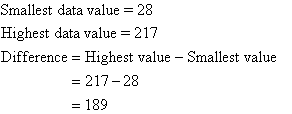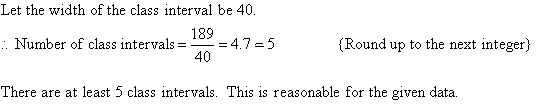Step 1:  Construct a table with three columns, and then write the data groups or class intervals in the first column.  The size of each group is 40.  So, the groups will start at 0, 40, 80, 120, 160 and 200 to include all of the data.  Note that in fact we need 6 groups (1 more than we first thought).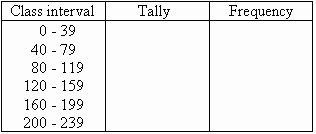Step 2:  Go through the list of data values.  For the first data value in the list, 28, place a tally mark against the group 0-39 in the second column.  For the second data value in the list, 122, place a tally mark against the group 120-159 in the second column.  For the third data value in the list, 217, place a tally mark against the group 200-239 in the second column.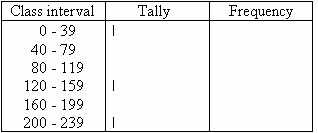We continue this process until all of the data values in the set are tallied.

Step 3:  Count the number of tally marks for each group and write it in the third column.  The finished frequency table is as follows: